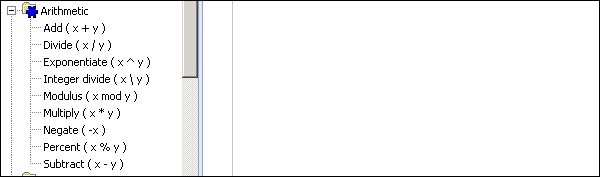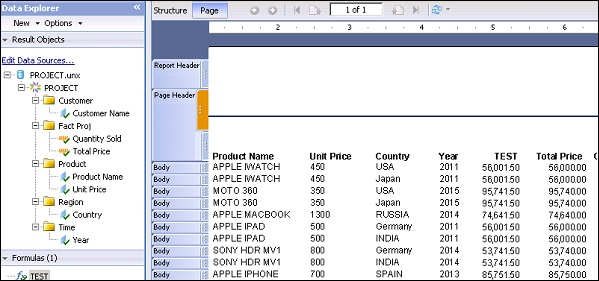# Crystal Reports - Applying Calculations

Calculated formulas are used to perform mathematical calculations in Crystal Reports and they can be designed in the formula workshop.

Arithmetic operators are used to design calculation formulas in workshop. There are different Arithmetic operators that can be used to Add, Divide, Exponential, Multiply, etc.To apply a calculation in the formula, drag the object from the objects pane to the formula text pane and use operators to perform the required calculation. Once you have saved the formula using the save option at the bottom, this formula will be saved under the Formula tab in Data explorer.You can then drag the formula to Report structure and go to Page tab to see the calculated data in the report.List of Arithmetic operators from highest to lowest precedence are −

• Exponentiation
• Negation
• Multiply, Divide and percentage
• Integer division
• Mod